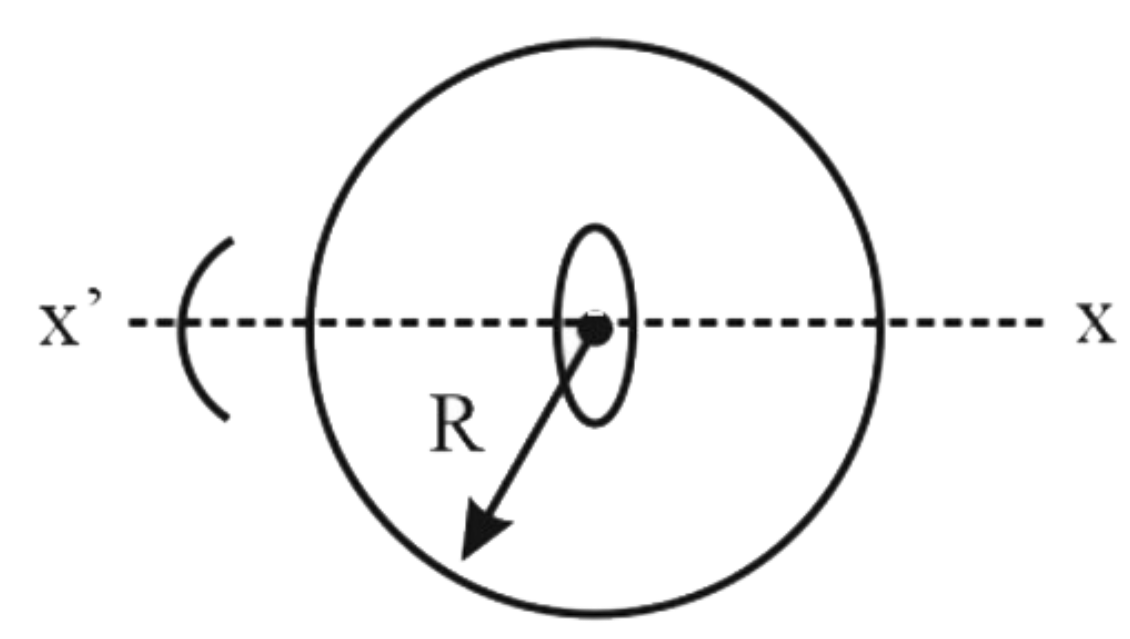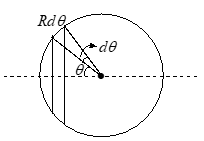A non – conducting ring of radius R having uniformly distributed charge Q starts rotating about X X’  axis passing through the  centre in the plane of the ring with an angular acceleration ‘α’ as shown in the figure.  Another small conducting ring having radius a(a<

# A non – conducting ring of radius R having uniformly distributed charge Q starts rotating about $\mathrm{X}\text{\hspace{0.17em}X'}$  axis passing through the  centre in the plane of the ring with an angular acceleration $\text{'}\alpha \text{'}$ as shown in the figure.  Another small conducting ring having radius $a\left(a< is kept fixed at the centre of bigger ring in such a way that the axis  is passing through its centre and perpendicular to its plane.  If the resistance of the small ring is $r=1\Omega$, find the induced current in it (in ampere).(Given  $Q=\frac{16×{10}^{2}}{{\mu }_{o}}C,R=1\mathrm{m},a=0.1\mathrm{m},\alpha =8\mathrm{rad}/{\mathrm{s}}^{-2}$)

Register to Get Free Mock Test and Study Material

+91

Verify OTP Code (required)

### Solution:

Magnetic Field on axis at center is given by,

$dB=\frac{{\mu }_{O}di\left(r{\right)}^{2}}{2{\left({r}^{2}+{x}^{2}\right)}^{3/2}}$

here $\text{r=Rsinθ}$ and $\sqrt{{\mathrm{r}}^{2}+{\mathrm{x}}^{2}}=\mathrm{R}$Register to Get Free Mock Test and Study Material

+91

Verify OTP Code (required)